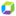cancel
Showing results for
Show  only  | Search instead for
Did you mean:

## How is the Apdex measure calculating?Organizer

Hello!

I understand how apdex is caluclated - [(num. of satisfied actions + (num of tolerating visits *0.5)) / total num of actions], but what I don't understand is the measure. This might be my misunderstanding of measures, but I thought they were calculated every 10 seconds. So, is the apdex measure evaluating the apdex score every 10 seconds? If I change my resolution to 1 hr, is it giving me the ave/max/min/last of every 10 second apdex score? If I use "last" and have my resolution set to 1 hr, will it give me the "real" apdex score or the apdex caluclation of the last 10 second slice?

Thanks so much!

1 REPLY 1Dynatrace Pro

This might be my misunderstanding of measures, but I thought they were calculated every 10 seconds. So, is the apdex measure evaluating the apdex score every 10 seconds
- I could be wrong here but I believe apdex is calculated at the end of every visit to give the most accurate statement

If I change my resolution to 1 hr, is it giving me the ave/max/min/last of every 10 second apdex score?
-Yes it will be looking at all measures within the last hour and calculating the avg/min/max of each score
If I use "last" and have my resolution set to 1 hr, will it give me the "real" apdex score or the apdex caluclation of the last 10 second slice?
- Last will give you whatever the very last segment was so in a set of .5,1,.75.25, my avg is .5 my max is 1 min is .25 and last is .25
Hope that helps